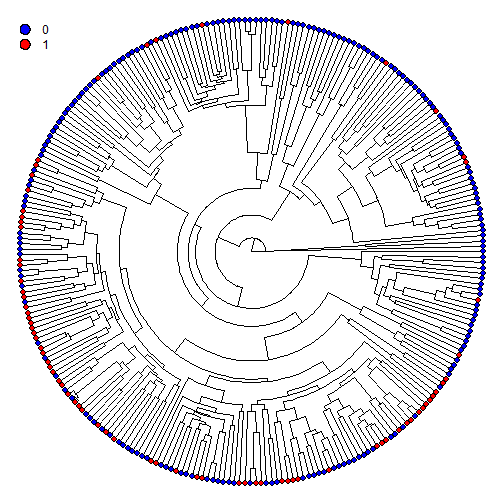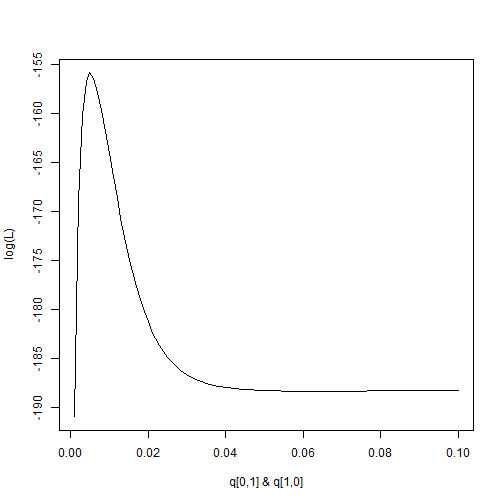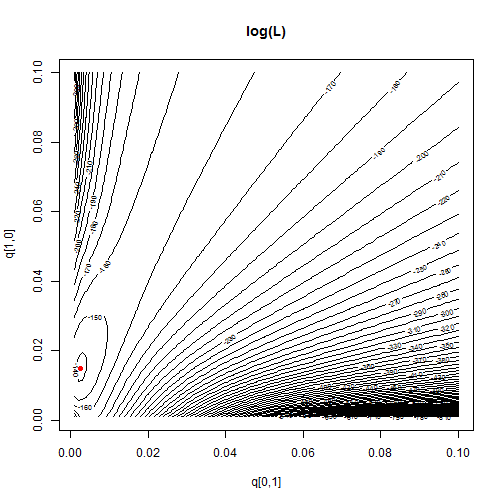## Tuesday, September 20, 2016

### Updates to `fitMk` to permit more user control of optimization

I just pushed some relatively small updates to the phytools function `fitMk`, which (aptly) fits the so-called Mk discrete character evolution model. The updates allow for more user control of optimization - specifically by allowing the user to specify an optimization method (`opt.method`), which can be `"nlminb"` or `"optim"`, and for the user to set initial values for the model parameters.

Note that `fitMk` takes a lot of code from the ape function `ace` (for ancestral character estimation); however `fitMk` allows the user to specify a fixed value of the transition matrix, `Q`, a non-flat prior probability density, `pi`, ambiguous states at the tips of the tree in the form of a matrix, and (now) an optimization method and starting values for the optimization. The function `fitDiscrete` in geiger is quite powerful for fitting this model as well.

These updates are in part inspired by a recent post I wrote about an interesting failure of optimization in the function. Since we are doing numerical optimization, not exhaustive enumeration, there is always a chance we will fail to find the ML solution! Hopefully these updates will allow users to be more confident that they have in fact converged on the tree MLEs for their model.

`fitMk` is also used internally by `make.simmap` and `rerootingMethod` (and perhaps other functions that I'm not thinking of now); however I have not yet migrated these options to these other methods.

Here's a demo with our dataset from before. This is a real empirical dataset, but one in which the taxon names have been obfuscated:

``````library(phytools)
packageVersion("phytools")
``````
``````##  '0.5.52'
``````
``````tree
``````
``````##
## Phylogenetic tree with 271 tips and 270 internal nodes.
##
## Tip labels:
##  t1, t2, t3, t4, t5, t6, ...
##
## Rooted; includes branch lengths.
``````
``````head(x,n=100); cat("....\n")
``````
``````##   t1   t2   t3   t4   t5   t6   t7   t8   t9  t10  t11  t12  t13  t14  t15
##    0    0    0    0    0    0    0    0    0    0    0    0    0    0    0
##  t16  t17  t18  t19  t20  t21  t22  t23  t24  t25  t26  t27  t28  t29  t30
##    0    1    1    0    0    0    0    0    0    0    0    0    1    0    0
##  t31  t32  t33  t34  t35  t36  t37  t38  t39  t40  t41  t42  t43  t44  t45
##    0    0    0    0    0    0    0    0    0    0    1    0    0    0    0
##  t46  t47  t48  t49  t50  t51  t52  t53  t54  t55  t56  t57  t58  t59  t60
##    0    0    0    0    0    0    0    0    0    0    0    0    0    0    0
##  t61  t62  t63  t64  t65  t66  t67  t68  t69  t70  t71  t72  t73  t74  t75
##    1    0    0    0    0    0    0    0    0    0    0    0    0    0    0
##  t76  t77  t78  t79  t80  t81  t82  t83  t84  t85  t86  t87  t88  t89  t90
##    0    1    0    0    0    0    0    0    0    0    1    0    1    0    0
##  t91  t92  t93  t94  t95  t96  t97  t98  t99 t100
##    0    0    0    0    0    0    0    0    1    0
``````
``````## ....
``````
``````plotTree(tree,type="fan",lwd=1,ftype="off")
tiplabels(pie=to.matrix(x[tree\$tip.label],c(0,1)),
piecol=c("blue","red"),cex=0.3)
c(0,1)),x=-176,y=169,prompt=FALSE,shape="circle")
``````OK, let's fit our model:

``````fit.nlminb<-fitMk(tree,x,opt.method="nlminb")
fit.nlminb
``````
``````## Object of class "fitMk".
##
## Fitted (or set) value of Q:
##           0         1
## 0 -1.000148  1.000148
## 1  1.000148 -1.000148
##
## Fitted (or set) value of pi:
##   0   1
## 0.5 0.5
##
## Log-likelihood: -187.842905
##
## Optimization method used was "nlminb"
``````

This was a rate that was about 200 × too high! Let's try the `"optim"` method for optimization. As you might guess, this substitutes the `optim` function for `nlminb` internally, and is a little bit slower as a consequence:

``````fit.optim<-fitMk(tree,x,opt.method="optim")
fit.optim
``````
``````## Object of class "fitMk".
##
## Fitted (or set) value of Q:
##           0         1
## 0 -0.005093  0.005093
## 1  0.005093 -0.005093
##
## Fitted (or set) value of pi:
##   0   1
## 0.5 0.5
##
## Log-likelihood: -155.900603
##
## Optimization method used was "optim"
``````

This gives us the correct answer this time; however it may not in general. For instance if we initial our transition rate with a value that is too high it will fail to converge on the correct solution:

``````fit.optim.q0.1<-fitMk(tree,x,opt.method="optim",q.init=0.1)
fit.optim.q0.1
``````
``````## Object of class "fitMk".
##
## Fitted (or set) value of Q:
##           0         1
## 0 -1.389036  1.389036
## 1  1.389036 -1.389036
##
## Fitted (or set) value of pi:
##   0   1
## 0.5 0.5
##
## Log-likelihood: -187.842886
##
## Optimization method used was "optim"
``````

Finally, as I showed before it is possible to visualize the likelihood surface for the transition rate. This is also possible in two dimensions for different forward & backward rates, q0,1 & q1,0. For instance:

``````## one dimension
q<-seq(0.001,0.1,by=0.001)
logL<-sapply(q,function(q,tree,x)
logLik(fitMk(tree,x,fixedQ=matrix(c(-q,q,q,-q),2,2))),
tree=tree,x=x)
plot(q,logL,type="l",xlab="q[0,1] & q[1,0]",ylab="log(L)")
````````````## two dimensions
logL<-sapply(q,function(q1,q2,tree,x) sapply(q2,
function(q2,q1,tree,x) logLik(fitMk(tree,x,fixedQ=
matrix(c(-q1,q1,q2,-q2),2,2))),tree=tree,x=x,q1=q1),
tree=tree,x=x,q2=q)
contour(logL,x=q,y=q,nlevels=100,xlab="q[0,1]",ylab="q[1,0]",
main="log(L)")
fitARD<-fitMk(tree,x,model="ARD")
points(fitARD\$rates,fitARD\$rates,col="red",pch=19)
``````Cool.